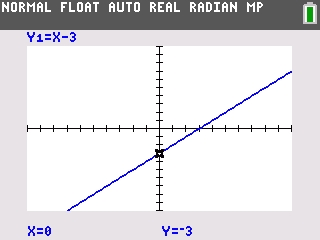# Knowledge Base

## Solution 34504: Graphing a Function on the TI-84 Plus C Silver Edition Graphing Calculator.

### How do I graph a function on a TI-84 Plus C Silver Edition graphing calculator?

The function graphing mode will graph equations in the format of y in terms of x. Therefore, equations will need to be solved in terms of y before being entered into the unit. The example below will demonstrate how to graph a function on a TI-84 Plus C Silver Edition graphing calculator.

Example:

Graph y - x = -3

Solution:

First, solve for y. This is done manually using algebra.

The new equation is: y=x-3

To graph the equation:

1) Press [Y=]
2) Input x - 3 on the top line after Y1=
3) Press [GRAPH]

The graph will now be displayed on the screen.